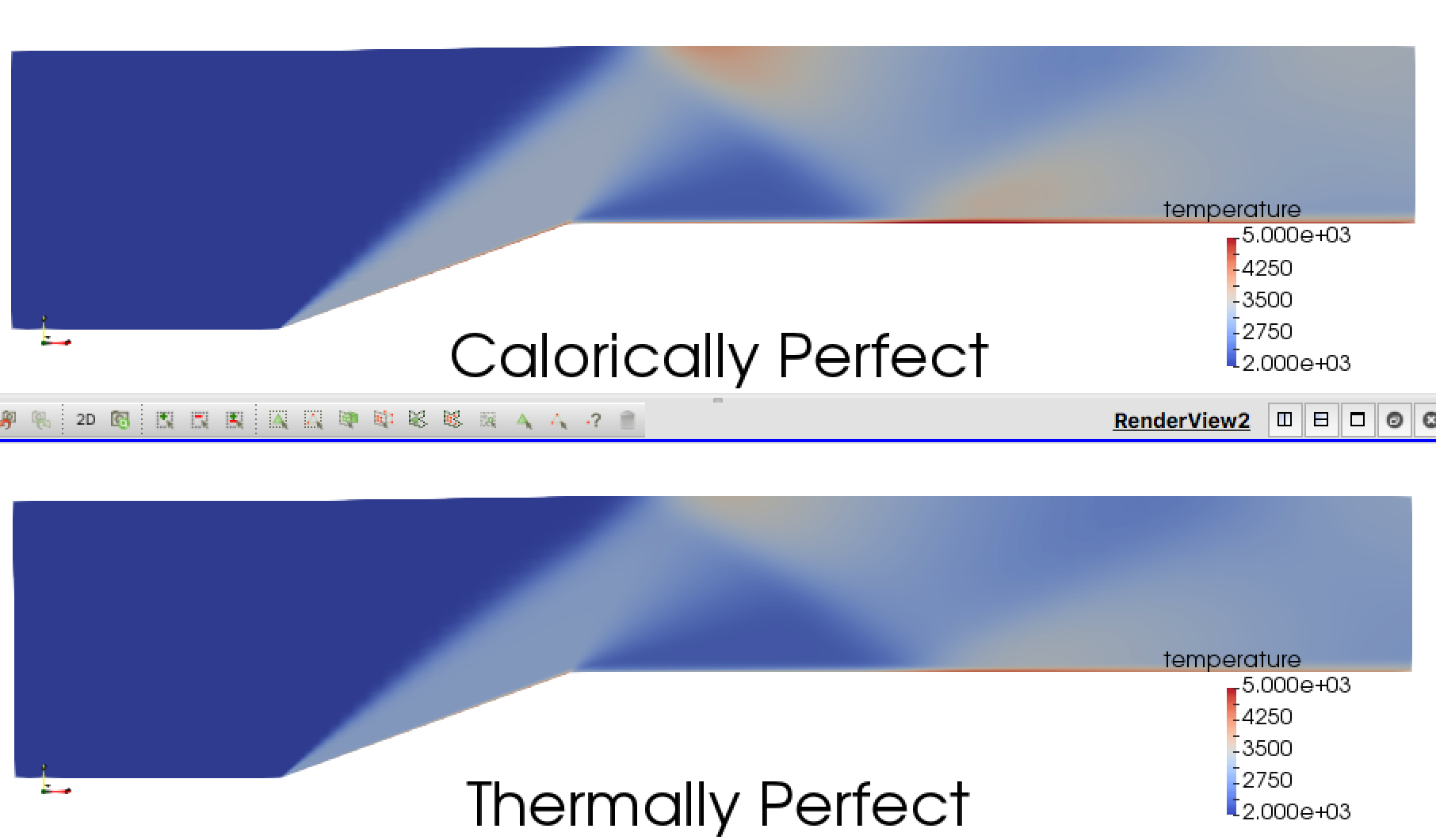## Calorically Perfect Gas

The default thermodynamic model in Aither is the calorically perfect gas model. Calorically perfect gases have constant specific heats $\left( c_p, c_v \right)$, and therefore a constant $\gamma$. In general the calorically perfect gas model is a good assumption for air at lower temperatures. The molecules of a calorically perfect gas are assumed to be rigid so there is no vibrational component of internal energy. The equations below show the implementation of the calorically perfect gas thermodynamic model in Aither.

## Thermally Perfect Gas

As the temperature of a gas increases the vibrational modes of its molecules are activated [1,2]. This means that some of the energy of the gas will move into these vibrational modes instead of raising the temperature of the gas. Therefore at higher temperatures where the vibrational modes are activated a thermally perfect gas will have a cooler temperature than a calorically perfect gas. The temperature at which the vibrational modes become significant is determined by the vibrational temperature $T_v$ of the gas. As the temperature of the gas approaches this value the vibrational component of internal energy becomes more and more significant.

The activiation of vibrational modes also means the the specific heats are no longer constant. Instead they are assumed to be functions of temperature only. The equations below show the implementation of the thermally perfect thermodynamic model in Aither.

In Aither the thermally perfect thermodynamic model can be activated as shown below.

fluids: <fluid(name=air; n=2.5; molarMass=0.02897; vibrationalTemperature=3056)>
thermodynamicModel: thermallyPerfect


## Example Problem

An example problem of when the thermally perfect model is needed is now a part of the test cases that come with the Aither repository. It is currently only available on the develop branch, but will be available on master after the next release. The test case involves hot supersonic flow over a 20 degree ramp. The freestream conditions of the flow are Mach 3, static temperature of 2000 K, and static pressure of 229,600 Pa.Comparison of calorically perfect and thermally perfect thermodynamic models.

The results show that behind the shocks the thermally perfect gas model predicts a cooler flow than the calorically perfect gas model. This is expected as with the thermally perfect model some of the energy goes into the vibrational modes of the gas molecules.

 Lampe, Dietrich Rudolf. “Thermally Perfect, Calorically Imperfect Taylor-Maccoll Flow”. 1994.

 Anderson, John. “Hypersonic and High Temperature Gas Dynamics”. 2nd Edition. AIAA. 2006.